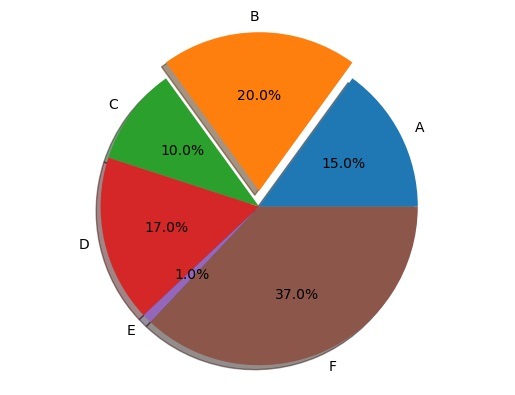# Python | Pie Chart with Shadow

Submitted by Anuj Singh, on August 01, 2020

A pie plot or a pie chart is a circular statistical graphic technique, in which a circle is divided into slices with respect to numerical proportion. In a pie chart, the arc length, central angle, and area of each slice, is proportional to the quantity it represents. The sum of the total is always equal to 100 percent in the basic pie chart.

The name pie in the pie plot is derived for its resemblance to a pie that has been sliced. Pie charts are very widely used in the different types of projects and business world. Matplotlib has a defined function in

matplotlib.pyplot.pie() for plotting a pie chart. In this tutorial, we are going to add shadow to the pie chart to create a fancy pie chart ready for presentations using an inbuilt command shadow='True'. The following example is an illustration of the same.## Python code for pie chart with shadow

```# Data Visualization using Python

import matplotlib.pyplot as plt

labels = 'A', 'B', 'C', 'D', 'E', 'F'
sizes = [15, 20, 10, 17, 1, 37]
explode = (0, 0.1, 0, 0, 0, 0)

plt.figure()
plt.axis('equal')
plt.show()
```

Output:

```Output is as figure
```